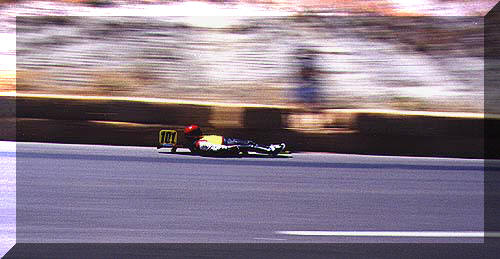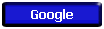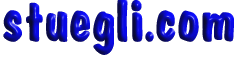#2.1 MOTION IS RELATIVE

Relative Motion
What does the phrase "motion is relative" mean? Find out in this online tutorial from the Department of Physics at the University of Illinois at Urbana-Champaign.
Foucault's Pendulum
Most of the time, the relative motion of the earth does not affect our perception of motion. However, in 1848, Leon Foucault discovered that when a large pendulum swings, the earth appears to "move under it." Learn more about Foucault's pendulum from Dr. Kruszelnicki's fact-filled site.

2.2 SPEED

Average vs. Instantaneous Speed What is the difference between average speed and instantaneous speed? This GIF animation from the Multimedia Physics Studios should clear up any confusion

2.3 VELOCITY

Velocity Learn all about velocity in this chapter from the Learn Physics Today online physics tutorial. It includes graphics, animation, and self-check questions.
Speed and Velocity What's the difference between speed and velocity? Find out by exploring this interactive tutorial from The Physics Classroom
Hot Wheels Track Check out the velocity and acceleration vectors of a Hot Wheels car as it moves around the race track in this GIF animation from the Multimedia Physics Studios

2.4 ACCELERATION

Accelerating Cars
Which car is accelerating? If necessary, review the definition of acceleration. animation from the Multimedia Physics Studios.
Accelerationarn all about acceleration in this chapter from theLearn Physics Today online physics tutorial. It includes graphics, animation, and self-check questions.

2.5 FREE FALL: HOW FAST

Free Fall and the Acceleration of Gravity Free fall is defined as "motion under the influence of gravitational force only." Find out more about free fall in this interactive tutorial from The Physics Classroom.

2.6 FREE FALL: HOW FAR

How fast? and How far? How fast something moves is entirely different from how far it moves. Learn more about fast, far, and free fall in this helpful interactive tutorial from The Physics Classroom

2.7 GRAPHS OF MOTION

Describing Motion with Position vs. Time Graphs Need help understanding position vs. time graphs? This is the place to go! You'll find everything you need in this interactive tutorial from The Physics Classroom. Topics include:
Describing Motion with Velocity vs. Time Graphs The place to go for help with velocity vs. time graphs. You'll find everything you need in this interactive tutorial from The Physics Classroom. Topics include:
The Meaning of Shape for a v-t Graph
The Meaning of Slope for a v-t Graph
Relating the Shape to the Motion
Determining the Slope on a v-t Graph
Determining the Area on a v-t Graph
Ticker Tape Motion This GIF animation from the Multimedia Physics Studios displays a ball moving across a ticker tape diagram with corresponding graphs of postion, velocity, and acceleration. Variations include:
Constant PositiveVelocity
Constant Negative Velocity
Positive Velocity with a Positive Acceleration
Positive Velocity with a Negative Acceleration
Negative Velocity with a Negative Acceleration
Negative Velocity with a Positive Acceleration
The Passing Lane Two cars race across the screen in this GIF animation from the Multimedia Physics Studios. Which car is accelerating? Look at the speed vs. time graph for help. Also check out the same animation featuring a position vs. time graph.
The Stop Light A car at a stoplight is passed by another car moving with constant velocity. If the car at the stoplight accelerates, can it catch the other car? A GIF animation from the Multimedia Physics Studios.
Two Stage Rocket A two stage rocket only has fuel for 10 seconds. What does its graph of motion look like after 20 seconds? A GIF animation from the Multimedia Physics Studios.

2.8 AIR RESISTANCE AND FALLING OBJECTS

The Elephant and The Feather Which falls faster in air, an elephant or a feather? What if there's no air resistance? Watch this GIF animation from the Multimedia Physics Studios.
Free Fall and Air Resistance
Learn more about air resistance and falling objects in this interactive tutorial from The Physics Classroom.

2.9 HOW FAST, HOW FAR, HOW QUICKLY HOW FAST CHANGES

Acceleration The often confusing concept of acceleration is broken down into simple rules of thumb in this helpful interactive tutorial from The Physics Classroom.Copyright © 2005 -  S. B. EglI